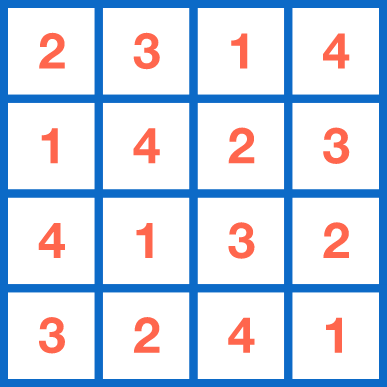# Infinite Latin Square?

Logic Level 1Is it possible to construct an infinite version of the table above (i.e. so that each row and each column contain all positive integers exactly once)?

×